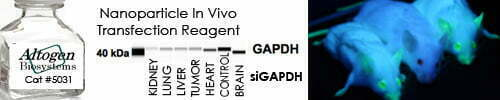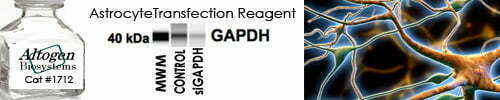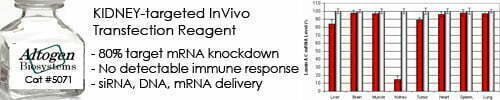••••Shop Products

# Common Scientific Constants

A reference chart of some common physical and mathematical constants.

 Physical  & Mathematical Constants Atmosphere (standard) atm 101.325 × 103 Pa Atomic mass unit (Dalton) amu 1.661 × 10-24 g Avogadro’s number N 6.022 × 1023/mol Base of natural log e 2.718 Becquerel Bq 1 dps Boltzmann constant k 1.381 × 10-23 J/K = 3.291 × 10-24 cal/K Curie Ci 3.7 × 1010 dps Electron volt eV 1.602 × 10-19 J = 3.828 × 10-20 cal Faraday constant F 96480 J/v • mol Gas constant R 1.987 cal/mol • K = 8.315 J/mol • K Gravitational constant G 6.674 × 10-11 Nm2/kg2 Gravity (standard) g 9.807 m/s2 Hubble constant H0 69.3 km/s/Mpc = 2.25 × 10-19 s-1 Natural logarithm ln x 2.303 log 10x Planck’s constant h 1.584 × 10-34 cal • s = 6.626 x 10-34 J • s pi π 3.1416 Speed of light (in vacuum) c 2.998 × 1010 cm/s

Sorry, comments are closed for this post.